# Standard deviation facts for kids

Kids Encyclopedia Facts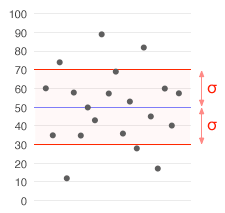A data set with a mean of 50 (shown in blue) and a standard deviation (σ) of 20.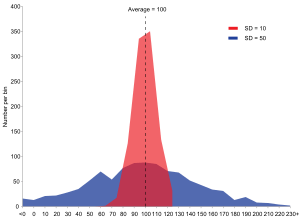Example of two sample populations with the same mean and different standard deviations. Red population has mean 100 and SD 10; blue population has mean 100 and SD 50.

Standard deviation is a number used to tell how measurements for a group are spread out from the average (mean or expected value). A low standard deviation means that most of the numbers are close to the average, while a high standard deviation means that the numbers are more spread out.

The reported margin of error is usually twice the standard deviation. Scientists commonly report the standard deviation of numbers from the average number in experiments. They often decide that only differences bigger than two or three times the standard deviation are important. Standard deviation is also useful in money, where the standard deviation on interest earned shows how different one person’s interest earned might be from the average.

Many times, only a sample, or part of a group can be measured. Then a number close to the standard deviation for the whole group can be found by a slightly different equation called the sample standard deviation, explained below. In which case, the standard deviation of the whole group is represented by the Greek letter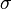$\sigma$, and that of the sample by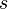$s$.

## Basic example

Consider a group having the following eight numbers: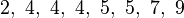$2,\ 4,\ 4,\ 4,\ 5,\ 5,\ 7,\ 9$

These eight numbers have the average (mean) of 5: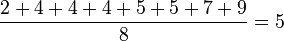$\frac{2 + 4 + 4 + 4 + 5 + 5 + 7 + 9}{8} = 5$

To calculate the population standard deviation, first find the difference of each number in the list from the mean. Then square the result of each difference: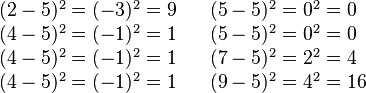$\begin{array}{lll} (2-5)^2 = (-3)^2 = 9 && (5-5)^2 = 0^2 = 0 \\ (4-5)^2 = (-1)^2 = 1 && (5-5)^2 = 0^2 = 0 \\ (4-5)^2 = (-1)^2 = 1 && (7-5)^2 = 2^2 = 4 \\ (4-5)^2 = (-1)^2 = 1 && (9-5)^2 = 4^2 = 16 \\ \end{array}$

Next, find the average of these values (sum divided by the number of numbers). Last, take the square root: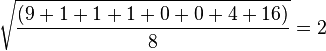$\sqrt{ \frac{(9 + 1 + 1 + 1 + 0 + 0 + 4 + 16)}{8} } = 2$

The answer is the population standard deviation. The formula is only true if the eight numbers we started with are the whole group. If they are only a part of the group picked at random, then we should use 7 (which is n − 1) instead of 8 (which is n) in the bottom (denominator) of the second-to-last step. Then the answer is the (biased corrected) sample standard deviation. This is called the Bessel's Correction.

## More examples

Here is a slightly harder, real-life example: The average height for grown men in the United States is 70", with a standard deviation of 3". A standard deviation of 3” means that most men (about 68%, assuming a normal distribution) have a height 3" taller to 3” shorter than the average (67"–73") — one standard deviation. Almost all men (about 95%) have a height 6” taller to 6” shorter than the average (64"–76") — two standard deviations. Three standard deviations include all the numbers for 99.7% of the sample population being studied. This is true if the distribution is normal (bell-shaped).

If the standard deviation were zero, then all men would be exactly 70" tall. If the standard deviation were 20", then some men would be much taller or much shorter than the average, with a typical range of about 50"–90".

For another example, each of the three groups {0, 0, 14, 14}, {0, 6, 8, 14} and {6, 6, 8, 8} has an average (mean) of 7. But their standard deviations are 7, 5, and 1. The third group has a much smaller standard deviation than the other two because its numbers are all close to 7. In general, the standard deviation tells us how far from the average the rest of the numbers tend to be, and it will have the same units as the numbers themselves. If, for example, the group {0, 6, 8, 14} is the ages of a group of four brothers in years, the average is 7 years and the standard deviation is 5 years.

Standard deviation may serve as a measure of uncertainty. In science, for example, the standard deviation of a group of repeated measurements helps scientists know how sure they are of the average number. When deciding whether measurements from an experiment agree with a prediction, the standard deviation of those measurements is very important. If the average number from the experiments is too far away from the predicted number (with the distance measured in standard deviations), then the theory being tested may not be right. For more information, see prediction interval.

### Application examples

Understanding the standard deviation of a set of values allows us to know how large a difference from the "average" (mean) is expected.

#### Weather

As a simple example, consider the average daily high temperatures for two cities, one inland and one near the ocean. It is helpful to understand that the range of daily high temperatures for cities near the ocean is smaller than for cities inland. These two cities may each have the same average daily high temperature. However, the standard deviation of the daily high temperature for the coastal city will be less than that of the inland city .

#### Sports

Another way of seeing it is to consider sports teams. In any sport, there will be teams that are good at some things and not at others. The teams that are ranked highest will not show a lot of differences in abilities. They do well in most categories. The lower the standard deviation of their ability in each category, the more balanced and consistent they are. Teams with a higher standard deviation, however, will be less predictable. A team that is usually bad in most categories will have a low standard deviation. A team that is usually good in most categories will also have a low standard deviation. However, a team with a high standard deviation might be the type of team that scores many points (strong offense) but also lets the other team score many points (weak defense).

Trying to know ahead of time which teams will win may include looking at the standard deviations of the various team "statistics." Numbers that are different from expected can match strengths vs. weaknesses to show what reasons may be most important in knowing which team will win.

In racing, the time a driver takes to finish each lap around the track is measured. A driver with a low standard deviation of lap times is more consistent than a driver with a higher standard deviation. This information can be used to help understand how a driver can reduce the time to finish a lap.

#### Money

In money, standard deviation may mean the risk that a price will go up or down (stocks, bonds, property, etc.). It can also mean the risk that a group of prices will go up or down (actively managed mutual funds, index mutual funds, or ETFs). Risk is one reason to make decisions about what to buy. Risk is a number people can use to know how much money they may earn or lose. As risk gets larger, the return on an investment can be more than expected (the "plus" standard deviation). However, an investment can also lose more money than expected (the "minus" standard deviation).

For example, a person had to choose between two stocks. Stock A over the past 20 years had an average return of 10 percent, with a standard deviation of 20 percentage points (pp). Stock B over the past 20 years had an average return of 12 percent but a higher standard deviation of 30 pp. Thinking about the risk, the person may decide that Stock A is the safer choice. Even though they may not make as much money, they probably will not lose much money either. The person may think that Stock B's 2 point higher average is not worth the additional 10 pp standard deviation (greater risk or uncertainty of the expected return).

### Rules for normally distributed numbers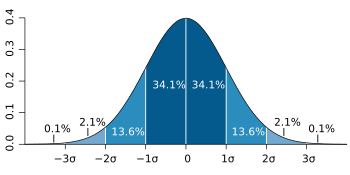Dark blue is less than one standard deviation from the mean. For the normal distribution, this includes 68.27 percent of the numbers; while two standard deviations from the mean (medium and dark blue) include 95.45 percent; three standard deviations (light, medium, and dark blue) include 99.73 percent; and four standard deviations account for 99.994 percent.

Most math equations for standard deviation assume that the numbers are normally distributed. This means that the numbers are spread out in a certain way on both sides of the average value. The normal distribution is also called a Gaussian distribution because it was discovered by Carl Friedrich Gauss. It is often called the bell curve because the numbers spread out to make the shape of a bell on a graph.

Numbers are not normally distributed if they are grouped on one side or the other side of the average value. Numbers can be spread out and still be normally distributed. The standard deviation tells how widely the numbers are spread out.

## Relationship between the average (mean) and standard deviation

The average (mean) and the standard deviation of a set of data are usually written together. Then a person can understand what the average number is and how widely other numbers in the group are spread out.

The way a group of numbers is spread out can also be given by the coefficient of variation (CV), which is the standard deviation divided by the average. It is a dimensionless number. Coefficient of variation is often multiplied by 100% and written as a percentage.

## History

The term standard deviation was first used in writing by Karl Pearson in 1894, after he used it in lectures. It was as a replacement for earlier names for the same idea: for example, Gauss used mean error.

## Images for kidsStandard deviation Facts for Kids. Kiddle Encyclopedia.